DOE Fundamentals Handbook - Nuclear Physics and Reactor TheoryDOE Fundamentals Handbook - Nuclear Physics and Reactor Theory

The Nuclear Physics and Reactor Theory Fundamentals Handbook contains elaborate discussions on Atomic and Nuclear Physics, Neutron Characteristics, Reactor Theory and Nuclear Parameters and the Theory of Reactor Operation. The information in the handbook is presented to provide a foundation for applying engineering concepts. It presents more than enough information to provide YOU with a fundamental knowledge level sufficient to really understand basic concepts of atomic physics, including the atomic nature of matter, Radioactive Decay, fission, the Neutron Life Cycle, Reactor Kinetics, as well as the interaction of radiation with matter and much, much more.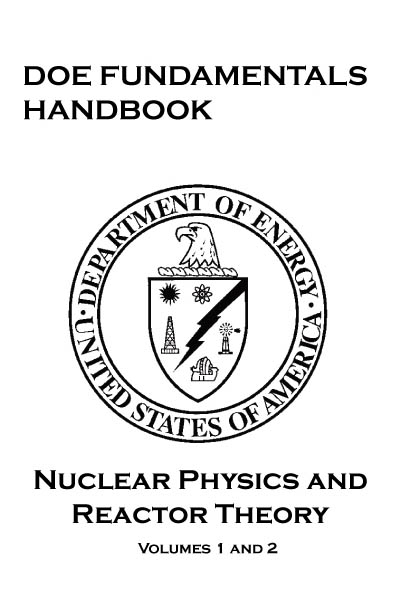VOLUMES 1-2

The Department of Energy (DOE) Fundamentals Handbooks consist of ten academic subjects, which include Mathematics; Classical Physics; Thermodynamics, Heat Transfer, and Fluid Flow; Instrumentation and Control; Electrical Science; Material Science; Mechanical Science; Chemistry; Engineering Symbology, Prints, and Drawings; and Nuclear Physics and Reactor Theory. The handbooks were first published as Reactor Operator Fundamentals Manuals in 1985 for use by DOE category A reactors. The subject areas, subject matter content, and level of detail of the Reactor Operator Fundamentals Manuals were determined from several sources and prepared by the DOE Training Coordination Program. Each handbook contains an abstract, a foreword, an overview, learning objectives, and text material, and is divided into modules.

The Nuclear Physics and Reactor Theory Handbook was originally developed to assist nuclear facility operating contractors provide operators, maintenance personnel, and the technical staff with the necessary fundamentals training to ensure a basic understanding of nuclear physics and reactor theory. The handbook includes information on the chart of the nuclides, neutron interactions, neutron flux, reaction rates, reactivity and reactivity coefficients, neutron poisons and subcritical multiplication. The Instrumentation and Control handbook presents more than enough information to provide the YOU with a fundamental knowledge level sufficient to understand the advanced theoretical concepts presented in other subject areas.

The Nuclear Physics and Reactor Theory handbook consists of four modules that are contained within two volumes. The following is a brief description of the information presented in each module of the handbook.

Module 1 - Atomic and Nuclear Physics

-Explanation- This module Introduces concepts of atomic physics including the atomic nature of matter, the chart of the nuclides, radioactivity and radioactive decay, neutron interactions and fission, and the interaction of radiation with matter.

ATOMIC NATURE OF MATTER

Structure of Matter Subatomic Particles Bohr Model of the AtomBohr Model of the Atom

The British physicist Ernest Rutherford postulated that the positive charge in an atom is concentrated in a small region called a nucleus at the center of the atom with electrons existing in orbits around it. Niels Bohr, coupling Rutherford's postulation with the quantum theory introduced by Max Planck, proposed that the atom consists of a dense nucleus of protons surrounded by electrons traveling in discrete orbits at fixed distances from the nucleus. An electron in one of these orbits or shells has a specific or discrete quantity of energy (quantum). When an electron moves from one allowed orbit to another allowed orbit, the energy difference between the two states is emitted or absorbed in the form of a single quantum of radiant energy called a photon. Figure 1 is Bohr's model of the hydrogen atom showing an electron as having just dropped from the third shell to the first shell with the emission of a photon that has an energy = hv. (h = Planck's constant = 6.63 x 10-34 J-s and v = frequency of the photon.) Bohr's theory was the first to successfully account for the discrete energy levels of this radiation as measured in the laboratory. Although Bohr's atomic model is designed specifically to explain the hydrogen atom, his theories apply generally to the structure of all atoms. Additional information on electron shell theory can be found in the Chemistry Fundamentals Handbook

CHART OF THE NUCLIDES

Chart of the NuclidesChart of the Nuclides

A tabulated chart called the Chart of the Nuclides lists the stable and unstable nuclides in addition to pertinent information about each one. Figure 3 shows a small portion of a typical chart. This chart plots a box for each individual nuclide, with the number of protons (Z) on the vertical axis and the number of neutrons (N = A - Z) on the horizontal axis.

The completely gray squares indicate stable isotopes. Those in white squares are artificially radioactive, meaning that they are produced by artificial techniques and do not occur naturally. By consulting a complete chart, other types of isotopes can be found, such as naturally occurring radioactive types (but none are found in the region of the chart that is illustrated in Figure 3).

Located in the box on the far left of each horizontal row is general information about the element. The box contains the chemical symbol of the element in addition to the average atomic weight of the naturally occurring substance and the average thermal neutron absorption cross section, which will be discussed in a later module. The known isotopes (elements with the same atomic number Z but different mass number A) of each element are listed to the right.

Mass Defect Binding Energy Energy Levels of Atoms Energy Levels of the NucleusEnergy Levels of the Nucleus

The nucleons in the nucleus of an atom, like the electrons that circle the nucleus, exist in shells that correspond to energy states. The energy shells of the nucleus are less defined and less understood than those of the electrons. There is a state of lowest energy (the ground state) and discrete possible excited states for a nucleus. Where the discrete energy states for the electrons of an atom are measured in eV or keV, the energy levels of the nucleus are considerably greater and typically measured in MeV.

A nucleus that is in the excited state will not remain at that energy level for an indefinite period. Like the electrons in an excited atom, the nucleons in an excited nucleus will transition towards their lowest energy configuration and in doing so emit a discrete bundle of electromagnetic radiation called a gamma ray (ã-ray). The only differences between x-rays and ã-rays are their energy levels and whether they are emitted from the electron shell or from the nucleus.

The ground state and the excited states of a nucleus can be depicted in a nuclear energy-level diagram. The nuclear energy-level diagram consists of a stack of horizontal bars, one bar for each of the excited states of the nucleus. The vertical distance between the bar representing an excited state and the bar representing the ground state is proportional to the energy level of the excited state with respect to the ground state. This difference in energy between the ground state and the excited state is called the excitation energy of the excited state. The ground state of a nuclide has zero excitation energy. The bars for the excited states are labeled with their respective energy levels. Figure 7 is the energy level diagram for nickel-60.

Stability of Nuclei Natural Radioactivity Nuclear Decay Alpha Decay (α) Beta Decay (β) Electron Capture (EC, K-capture) Gamma Emission (γ) Internal Conversion Isomers and Isomeric Transition Decay Chains Predicting Type of DecayPredicting Type of Decay

Radioactive nuclides tend to decay in a way that results in a daughter nuclide that lies closer to the line of stability. Due to this, it is possible to predict the type of decay that a nuclide will undergo based on its location relative to the line of stability on the Chart of the Nuclides.

Figure 9 illustrates the type of decay nuclides in different regions of the chart will typically undergo. Nuclides that are below and to the right of the line of stability will usually undergo (3- decay. Nuclides that are above and to the left of the line of stability will usually undergo either R+ decay or electron capture. Most nuclides that will undergo a decay are found in the upper right hand region of the chart. These are general rules that have many exceptions, especially in the region of the heavy nuclides.

Summary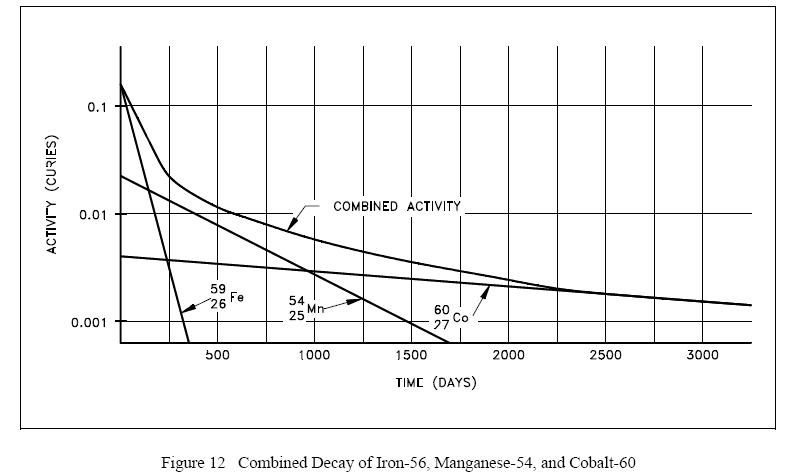Plotting the manner in which the activities of each of the three nuclides decay over time demonstrates that initially the activity of the shortest-lived nuclide (iron-59) dominates the total activity, then manganese-54 dominates. After almost all of the iron and manganese have decayed away, the only contributor to activity will be the cobalt-60. A plot of this combined decay is shown in Figure 12.

NEUTRON INTERACTIONS

Scattering Elastic ScatteringFigure 16 illustrates the process of elastic scattering of a neutron off a target nucleus. In the elastic scattering reaction, the conservation of momentum and kinetic energy is represented by the equations below. ½

Conservation of momentum ( mv )

( mn vn,i ) + ( mT vT,i ) = ( mn vn,f ) + ( mT vT,f )

Conservativation of kinetic energy ( ½ m v2 )

( ½ mn v2n,i ) + ( ½ mT v2n,f ) + ( ½mT v2T,f )

NUCLEAR FISSION

Fission Liquid Drop Model of a NucleusReferring to Figure 18(A), the nucleus in the ground state is undistorted, and its attractive nuclear forces are greater than the repulsive electrostatic forces between the protons within the nucleus. When an incident particle (in this instance a neutron) is absorbed by the target nucleus, a compound nucleus is formed. The compound nucleus temporarily contains all the charge and mass involved in the reaction and exists in an excited state. The excitation energy added to the compound nucleus is equal to the binding energy contributed by the incident particle plus the kinetic energy possessed by that particle. Figure 18(B) illustrates the excitation energy thus imparted to the compound nucleus, which may cause it to oscillate and become distorted. If the excitation energy is greater than a certain critical energy, the oscillations may cause the compound nucleus to become dumbbell-shaped. When this happens, the attractive nuclear forces (short-range) in the neck area are small due to saturation, while the repulsive electrostatic forces (long-range) are only slightly less than before. When the repulsive electrostatic forces exceed the attractive nuclear forces, nuclear fission occurs, as illustrated in Figure 18(C).

Module 2 - Reactor Theory (Nuclear Parameters)

-Explanation- This module provides information on reactor theory and neutron characteristics. Includes topics such as neutron sources, neutron flux, neutron cross sections, reaction rates, neutron moderation, and prompt and delayed neutrons.

NEUTRON SOURCES

Neutron Sources Intrinsic Neutron SourcesA limited number of neutrons will always be present, even in a reactor core that has never been operated, due to spontaneous fission of some heavy nuclides that are present in the fuel. Uranium-238, uranium-235, and plutonium-239 undergo spontaneous fission to a limited extent. Uranium-238, for example, yields almost 60 neutrons per hour per gram. Table 1 illustrates a comparison of the rate at which different heavy nuclides produce neutrons by spontaneous fission. Californium-252 is not an intrinsic neutron source, but will be discussed in the section on installed neutron sources.

Another intrinsic neutron source is a reaction involving natural boron and fuel. In some reactors, natural boron is loaded into the reactor core as a neutron absorber to improve reactor control or increase core life-time. Boron-11 (80.1% of natural boron) undergoes a reaction with the alpha particle emitted by the radioactive decay of heavy nuclides in the fuel to yield a neutron as shown below.

NUCLEAR CROSS SECTIONS AND NEUTRON FLUX

Introduction Atom Density Cross SectionsThe variation of absorption cross sections with neutron energy is often complicated. For many elements the absorption cross sections are small, ranging from a fraction of a barn to a few barns for slow (or thermal) neutrons.

For a considerable number of nuclides of moderately high (or high) mass numbers, an examination of the variation of the absorption cross section with the energy of the incident neutron reveals the existence of three regions on a curve of absorption cross section versus neutron energy. This cross section is illustrated in Figure 1. First, the cross section decreases steadily with increasing neutron energy in a low energy region, which includes the thermal range (E < 1 eV). In this region the absorption cross section, which is often high, is inversely proportional to the velocity (v). This region is frequently referred to as the "1/v region," because the absorption cross section is proportional to 1/v, which is the reciprocal of neutron velocity. Following the 1/v region, there occurs the "resonance region" in which the cross sections rise sharply to high values called "resonance peaks" for neutrons of certain energies, and then fall again. These energies are called resonance energies and are a result of the affinity of the nucleus for neutrons whose energies closely match its discrete, quantum energy levels. That is, when the binding energy of a neutron plus the kinetic energy of the neutron are exactly equal to the amount required to raise a compound nucleus from its ground state to a quantum level, resonance absorption occurs.

NEUTRON FLUX SPECTRUM

Prompt Neutron Energies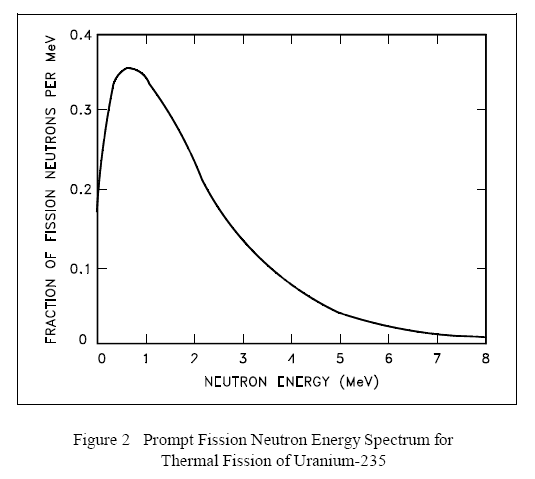Prompt Neutron Energies

The neutrons produced by fission are high energy neutrons, and almost all fission neutrons have energies between 0.1 MeV and 10 MeV. The neutron energy distribution, or spectrum, may best be described by plotting the fraction of neutrons per MeV as a function of neutron energy, as shown in Figure 2. From this figure it can be seen that the most probable neutron energy is about 0.7 MeV. Also, from this data it can be shown that the average energy of fission neutrons is about 2 MeV. Figure 2 is the neutron energy spectrum for thermal fission in uranium-235. The values will vary slightly for other nuclides.

Module 3 - Reactor Theory (Nuclear Parameters)

-Explanation- This module explains the nuclear parameters associated with reactor theory. Topics include the neutron life cycle, reactivity and reactivity coefficients, neutron poisons, and control rods.

NEUTRON LIFE CYCLE

Infinite Multiplication Factor, k. Four Factor Formula Fast Fission Factor, (e) Resonance Escape Probability, (p) Thermal Utilization Factor, (f) Reproduction Factor, (η) Effective Multiplication Factor Fast Non-Leakage Probability (L) Thermal Non-Leakage Probability (L) Six Factor Formula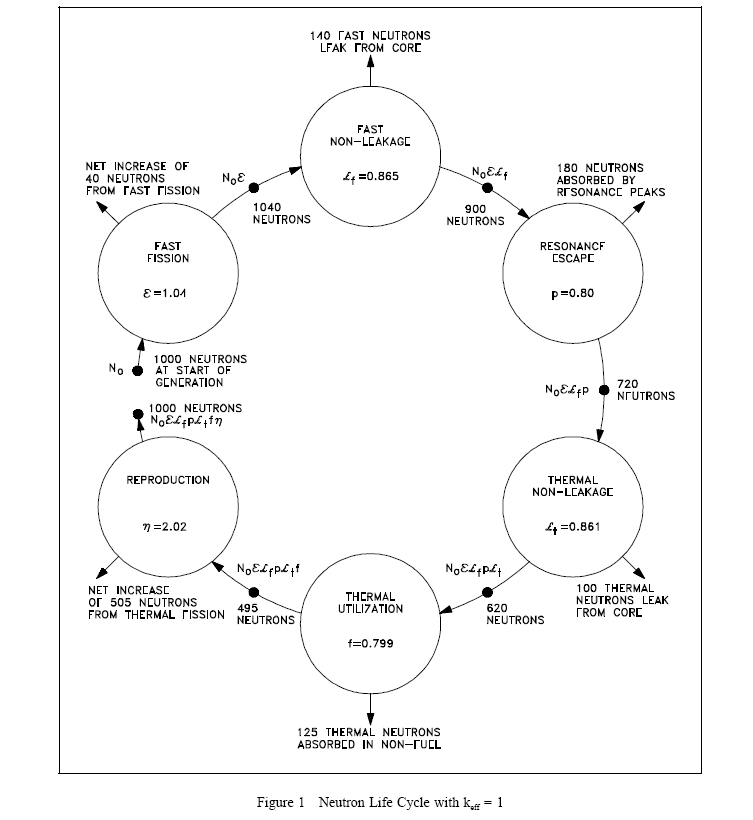REACTIVITY COEFFICIENTS

Moderator EffectsThe moderating ratio is merely the ratio of slowing down power to the macroscopic absorption cross section. The higher the moderating ratio, the more effectively the material performs as a moderator.

Another ratio, the moderator-to-fuel ratio (Nm/Nu), is very important in the discussion of moderators. As the reactor designer increases the amount of moderator in the core (that is, Nm/Nu increases), neutron leakage decreases. Neutron absorption in the moderator (Σm) increases and causes a decrease in the thermal utilization factor. Having insufficient moderator in the core (that is, Nm/Nu decreases) causes an increase in slowing down time and results in a greater loss of neutrons by resonance absorption. This also causes an increase in neutron leakage. The effects of varying the moderator-to-fuel ratio on the thermal utilization factor and the resonance probability are shown in Figure 2.

XENON

Fission Product Poisons Production and Removal of Xenon-135 Xenon-135 Response to Reactor ShutdownBecause the decay rate of iodine-135 is faster than the decay rate of xenon-135, the xenon concentration builds to a peak. The peak is reached when the product of the terms λINI is equal to λXeNXe (in about 10 to 11 hours). Subsequently, the production from iodine decay is less than the removal of xenon by decay, and the concentration of xenon-135 decreases. The greater the flux level prior to shutdown, the greater the concentration of iodine-135 at shutdown; therefore, the greater the peak in xenon-135 concentration after shutdown. This phenomenon can be seen in Figure 5, which illustrates the negative reactivity value of xenon-135 following shutdown from various neutron flux levels.

SAMARIUM AND OTHER FISSION PRODUCT POISONS

Production and Removal of Samarium-149 Samarium-149 Response to Reactor Shutdown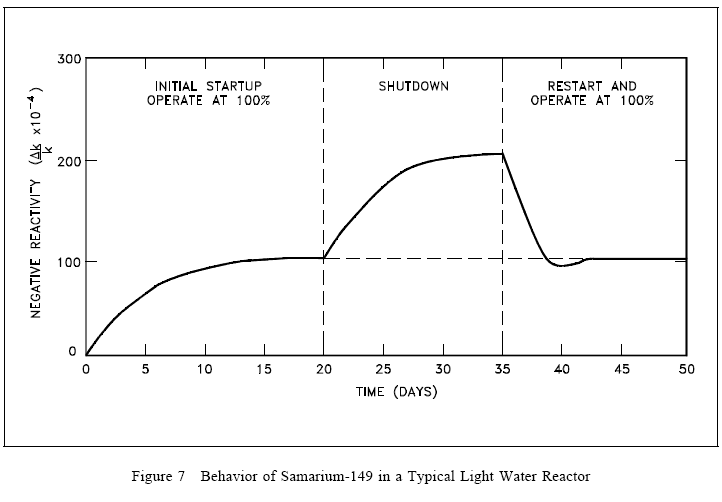Because samarium-149 is not radioactive and is not removed by decay, it presents problems somewhat different from those encountered with xenon-135, as illustrated in Figure 7. The equilibrium concentration and the poisoning effect build to an equilibrium value during reactor operation. This equilibrium is reached in approximately 20 days (500 hours), and since samarium-149 is stable, the concentration remains essentially constant during reactor operation. When the reactor is shutdown, the samarium-149 concentration builds up as a result of the decay of the accumulated promethium-149. The buildup of samarium-149 after shutdown depends upon the power level before shutdown. Samarium-149 does not peak as xenon-135 does, but increases slowly to a maximum value as shown in Figure 7. After shutdown, if the reactor is then operated at power, samarium-149 is burned up and its concentration returns to the equilibrium value. Samarium poisoning is minor when compared to xenon poisoning. Although samarium-149 has a constant poisoning effect during long-term sustained operation, its behavior during initial startup and during post-shutdown and restart periods requires special considerations in reactor design.

The xenon-135 and samarium-149 mechanisms are dependent on their very large thermal neutron cross sections and only affect thermal reactor systems. In fast reactors, neither these nor any other fission products have a major poisoning influence.

CONTROL RODS

Election of Control Rod Materials Types of Control Rods Control Rod Effectiveness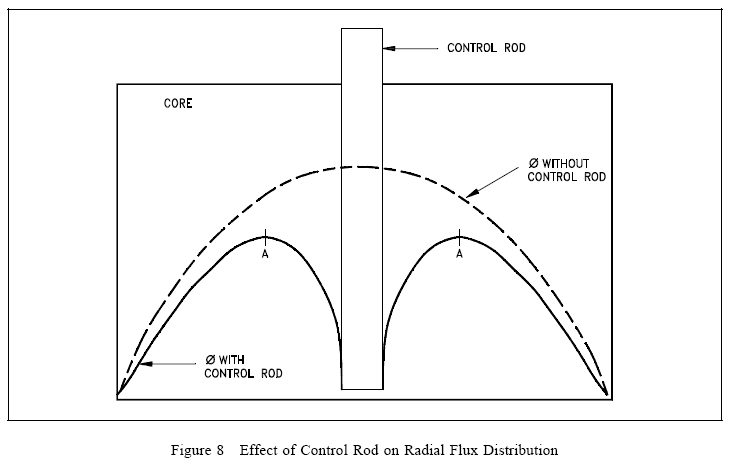Control Rod Effectiveness

The effectiveness of a control rod depends largely upon the value of the ratio of the neutron flux at the location of the rod to the average neutron flux in the reactor. The control rod has maximum effect (inserts the most negative reactivity) if it is placed in the reactor where the flux is maximum. If a reactor has only one control rod, the rod should be placed in the center of the reactor core. The effect of such a rod on the flux is illustrated in Figure 8.
If additional rods are added to this simple reactor, the most effective location is where the flux is maximum, that is, at point A. Numerous control rods are required for a reactor that has a large amount of excess reactivity (that amount of reactivity in excess of that needed to be critical). The exact amount of reactivity that each control rod inserts depends upon the reactor design. The change in reactivity caused by control rod motion is referred to as control rod worth.

Module 4 - Reactor Theory (Reactor Operations)

-Explanation- This module introduces the reactor operations aspect of reactor theory. Topics include subcritical multiplication, reactor kinetics, and reactor operation.

REACTOR KINETICS

Reactor Period (τ) Effective Delayed Neutron Fraction Effective Delayed Neutron Precursor Decay Constant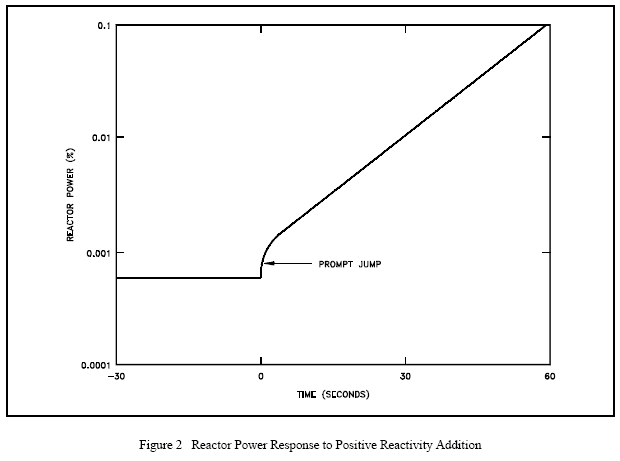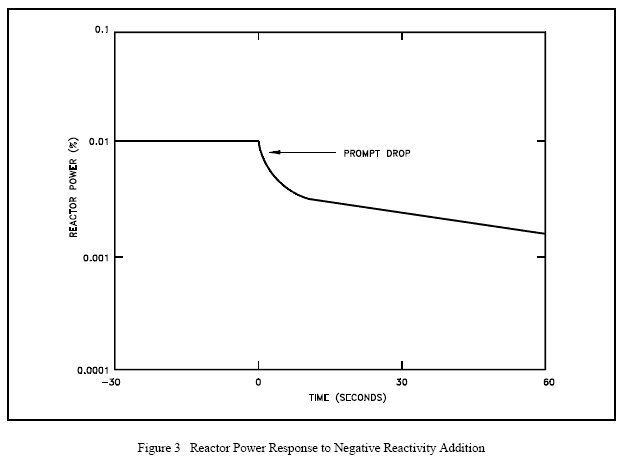If the positive reactivity added is less than the value of eff, the emission of prompt fission neutrons alone is not sufficient to overcome losses to non-fission absorption and leakage. If delayed neutrons were not being produced, the neutron population would decrease as long as the reactivity of the core has a value less than the effective delayed neutron fraction. The positive reactivity insertion is followed immediately by a small immediate power increase called the prompt jump. This power increase occurs because the rate of production of prompt neutrons changes abruptly as the reactivity is added. Recall from an earlier module that the generation time for prompt neutrons is on the order of 10-13 seconds. The effect can be seen in Figure 2. After the prompt jump, the rate of change of power cannot increase any more rapidly than the built-in time delay the precursor half-lives allow. Therefore, the power rise is controllable, and the reactor can be operated safely.

Conversely, in the case where negative reactivity is added to the core there will be a prompt drop in reactor power. The prompt drop is the small immediate decrease in reactor power caused by the negative reactivity addition. The prompt drop is illustrated in Figure 3. After the prompt drop, the rate of change of power slows and approaches the rate determined by the delayed term of Equation (4-7).

REACTOR OPERATION

Startup Estimated Critical Position Core Power Distribution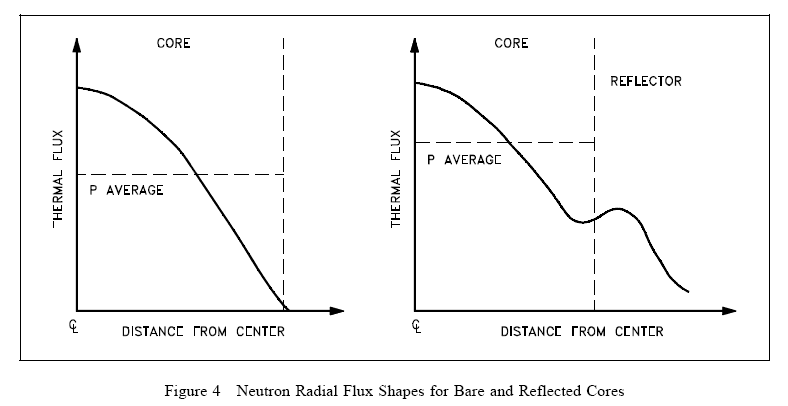Figure 4 shows the general effect of reflection in the thermal reactor system where core power is proportional to the thermal flux. Notice that a reflector can raise the power density of the core periphery and thus increase the core average power level without changing the peak power. As illustrated in Figure 4, the thermal flux in the reflector may actually be higher than that in the outermost fuel since there are very few absorptions in the reflectorVarying the fuel enrichment or fuel concentrations in the core radially, axially, or both, can readily be used to control power distribution. The simplified example illustrated in Figure 5 shows the effect of using a higher enrichment in the outer regions of the core. Varying fuel concentrations or poison loading for flux shaping is frequently referred to as zoning. In the example illustrated the large central peak is reduced, but the average power level remains the same.

Power Tilt Shutdown Margin Operation Temperature Pressure Power Level Flow Core Burnup Shutdown Decay Heat SummaryDOE Fundamentals Handbook - Nuclear Physics and Reactor Theory

The Nuclear Physics and Reactor Theory Fundamentals Handbook contains elaborate discussions on Atomic and Nuclear Physics, Neutron Characteristics, Reactor Theory and Nuclear Parameters and the Theory of Reactor Operation. The information in the handbook is presented to provide a foundation for applying engineering concepts. It presents more than enough information to provide YOU with a fundamental knowledge level sufficient to really understand basic concepts of atomic physics, including the atomic nature of matter, Radioactive Decay, fission, the Neutron Life Cycle, Reactor Kinetics, as well as the interaction of radiation with matter and much, much more.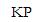About Us | Site Map | Mail Order Instructions | Contact Us | Press | ©2007 KnowledgePublications.com